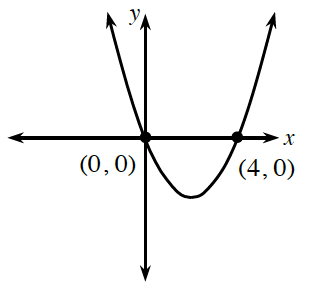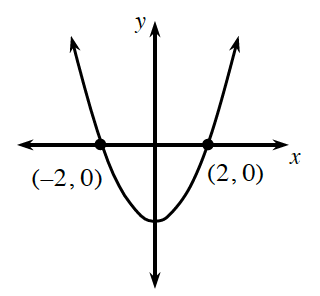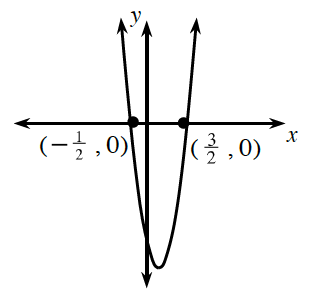### Home > CCA2 > Chapter C > Lesson C.1.3 > ProblemC-53

C-53.

Based on the graph, give a possible equation for the parabola.

For additional help, see problem 11-13.

1.The $x$-coordinates of the $x$-intercepts are roots of the equation. If you know the roots, can you find the factors?

The factors are $(x−0)$ and $(x−4)$.

$y=x(x−4)$

1.See the hints in part (a).

1.Instead of using fractions, multiply each factor by the fraction's denominator.

The factors can be written as
$(2x+1)$ and $(2x−3)$.